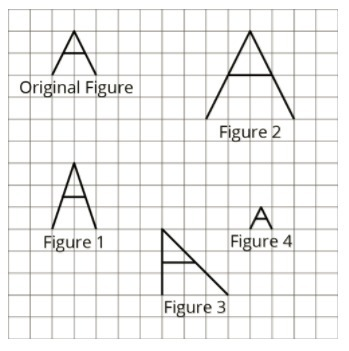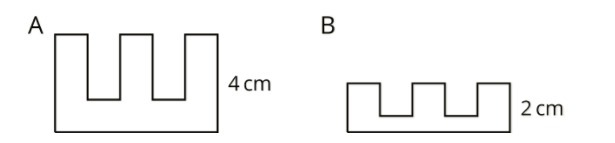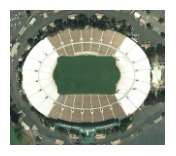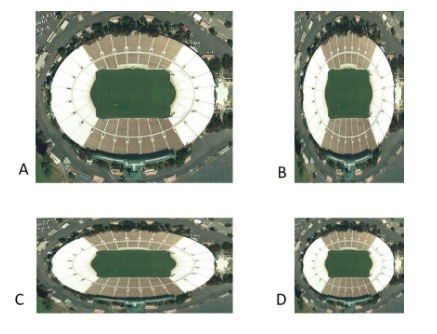PSA6N9K - Practice Problems---7.1 Lesson 1: What are Scaled Copies? (7.G.A.1 )
Part A)

Here is a figure that looks like the letter A, along with several other figures. Which figures are scaled copies of the original A?Select all that apply:
Part B)

Explain.

Part A)

Tyler says that Figure B is a scaled copy of Figure A because all of the peaks are half as tall.

Do you agree with Tyler?Select one:
Part B)

Explain.

Here is a picture of the Rose Bowl Stadium in Pasadena, CA.Here are some copies of the picture. Select all the pictures that are scaled copies of the original picture.Select all that apply:
Part A)

Complete the equation with a number that makes it true.

5______=15

Type your answer below as a number (example: 5, 3.1, 4 1/2, or 3/2):
Part B)

Complete the equation with a number that makes it true.

4⋅______=32

Type your answer below as a number (example: 5, 3.1, 4 1/2, or 3/2):
Part C)

Complete the equation with a number that makes it true.

6⋅______=9